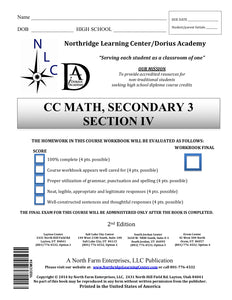# CC Math, Secondary 3, Section IV

Regular price
\$56.50
Regular price
Sale price
\$56.50
Unit price
per
Availability
Sold out

Common Core Mathematics, Secondary III 07-08-0000-100

The Common Core Math series culminates with this course. Building on knowledge from previous years, this course offers a rigorous approach towards mathematics that prepares students for collegiate math. This course begins with an introduction to statistics, teaching analysis of data sets through methods such as standard deviation and bell curve graphs, and finishes with graphing and analyzing spatial and logarithmic functions. Throughout the span of this course, students will learn how to multiply and divide complex polynomials; solve advanced trigonometric equations such as identity problems; and graph quadratic, exponential, logarithmic, absolute value, and trigonometric equations based on parent function relationships.

30-Day Extension - Click Here